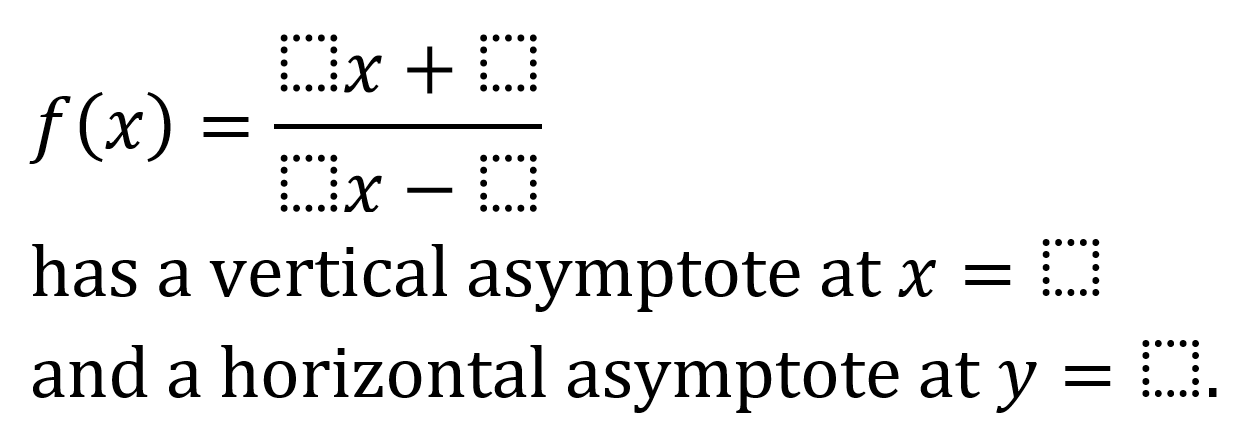# Asymptotes of Rational Functions

Directions: Using the digits 1 to 9 at most one time each, place a digit in each box create a true statement.### Hint

How do you determine the vertical asymptote of a rational function, and why does that happen? How do you determine the horizontal asymptote of a rational function, and why does that happen?

There are multiple answers. Here are two examples:

f(x) = (8x+1)/(2x-6) has a vertical asymptote at x = 3 and a horizontal asymptote at y = 4.

f(x) = (6x+7)/(2x-8) has a vertical asymptote at x = 4 and a horizontal asymptote at y = 3.

Source: Dana Harrington

## Evaluating Logs

Directions: Using the digits 1 to 9 at most one time each, place a digit …